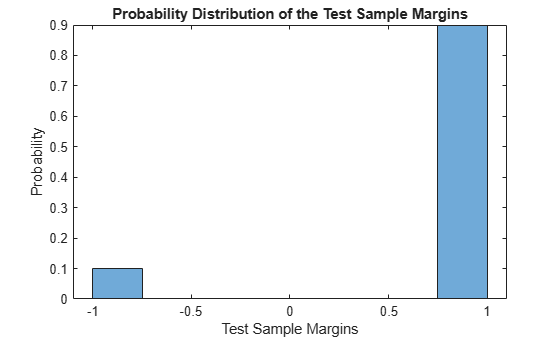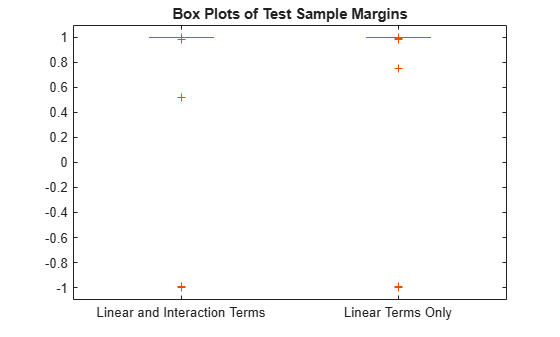# edge

Classification edge for generalized additive model (GAM)

## Syntax

``e = edge(Mdl,Tbl,ResponseVarName)``
``e = edge(Mdl,Tbl,Y)``
``e = edge(Mdl,X,Y)``
``e = edge(___,Name,Value)``

## Description

````e = edge(Mdl,Tbl,ResponseVarName)` returns the Classification Edge (`e`) for the generalized additive model `Mdl` using the predictor data in `Tbl` and the true class labels in `Tbl.ResponseVarName`.```
````e = edge(Mdl,Tbl,Y)` uses the predictor data in table `Tbl` and the true class labels in `Y`.```

example

````e = edge(Mdl,X,Y)` uses the predictor data in matrix `X` and the true class labels in `Y`.```

example

````e = edge(___,Name,Value)` specifies options using one or more name-value arguments in addition to any of the input argument combinations in previous syntaxes. For example, you can specify observation weights and whether to include interaction terms in computations.```

## Examples

collapse all

Estimate the test sample classification margins and edge of a generalized additive model. The test sample margins are the observed true class scores minus the false class scores, and the test sample edge is the mean of the margins.

Load the `fisheriris` data set. Create `X` as a numeric matrix that contains two sepal and two petal measurements for versicolor and virginica irises. Create `Y` as a cell array of character vectors that contains the corresponding iris species.

```load fisheriris inds = strcmp(species,'versicolor') | strcmp(species,'virginica'); X = meas(inds,:); Y = species(inds,:);```

Randomly partition observations into a training set and a test set with stratification, using the class information in `Y`. Specify a 30% holdout sample for testing.

```rng('default') % For reproducibility cv = cvpartition(Y,'HoldOut',0.30);```

Extract the training and test indices.

```trainInds = training(cv); testInds = test(cv);```

Specify the training and test data sets.

```XTrain = X(trainInds,:); YTrain = Y(trainInds); XTest = X(testInds,:); YTest = Y(testInds);```

Train a GAM using the predictors `XTrain` and class labels `YTrain`. A recommended practice is to specify the class names.

`Mdl = fitcgam(XTrain,YTrain,'ClassNames',{'versicolor','virginica'});`

`Mdl` is a `ClassificationGAM` model object.

Estimate the test sample classification margins and edge.

```m = margin(Mdl,XTest,YTest); e = edge(Mdl,XTest,YTest)```
```e = 0.8000 ```

Display the histogram of the test sample classification margins.

```histogram(m,length(unique(m)),'Normalization','probability') xlabel('Test Sample Margins') ylabel('Probability') title('Probability Distribution of the Test Sample Margins')```Estimate the test sample weighted edge (the weighted average of margins) of a generalized additive model.

Load the `fisheriris` data set. Create `X` as a numeric matrix that contains two sepal and two petal measurements for versicolor and virginica irises. Create `Y` as a cell array of character vectors that contains the corresponding iris species.

```load fisheriris idx1 = strcmp(species,'versicolor') | strcmp(species,'virginica'); X = meas(idx1,:); Y = species(idx1,:);```

Suppose that the quality of some measurements is lower because they were measured with older technology. To simulate this effect, add noise to a random subset of 20 measurements.

```rng('default') % For reproducibility idx2 = randperm(size(X,1),20); X(idx2,:) = X(idx2,:) + 2*randn(20,size(X,2));```

Randomly partition observations into a training set and a test set with stratification, using the class information in `Y`. Specify a 30% holdout sample for testing.

`cv = cvpartition(Y,'HoldOut',0.30);`

Extract the training and test indices.

```trainInds = training(cv); testInds = test(cv);```

Specify the training and test data sets.

```XTrain = X(trainInds,:); YTrain = Y(trainInds); XTest = X(testInds,:); YTest = Y(testInds);```

Train a GAM using the predictors `XTrain` and class labels `YTrain`. A recommended practice is to specify the class names.

`Mdl = fitcgam(XTrain,YTrain,'ClassNames',{'versicolor','virginica'});`

`Mdl` is a `ClassificationGAM` model object.

Estimate the test sample edge.

`e = edge(Mdl,XTest,YTest)`
```e = 0.8000 ```

The average margin is approximately 0.80.

One way to reduce the effect of the noisy measurements is to assign them less weight than the other observations. Define a weight vector that gives the higher quality observations twice the weight of the other observations.

```n = size(X,1); weights = ones(size(X,1),1); weights(idx2) = 0.5; weightsTrain = weights(trainInds); weightsTest = weights(testInds);```

Train a GAM using the predictors `XTrain`, class labels `YTrain`, and weights `weightsTrain`.

```Mdl_W = fitcgam(XTrain,YTrain,'Weights',weightsTrain,... 'ClassNames',{'versicolor','virginica'});```

Estimate the test sample weighted edge using the weighting scheme.

`e_W = edge(Mdl_W,XTest,YTest,'Weights',weightsTest)`
```e_W = 0.8770 ```

The weighted average margin is approximately 0.88. This result indicates that, on average, the labels from weighted classifier labels have higher confidence.

Compare a GAM with linear terms to a GAM with both linear and interaction terms by examining the test sample margins and edge. Based solely on this comparison, the classifier with the highest margins and edge is the best model.

Load the `ionosphere` data set. This data set has 34 predictors and 351 binary responses for radar returns, either bad (`'b'`) or good (`'g'`).

`load ionosphere`

Randomly partition observations into a training set and a test set with stratification, using the class information in `Y`. Specify a 30% holdout sample for testing.

```rng('default') % For reproducibility cv = cvpartition(Y,'Holdout',0.30);```

Extract the training and test indices.

```trainInds = training(cv); testInds = test(cv);```

Specify the training and test data sets.

```XTrain = X(trainInds,:); YTrain = Y(trainInds); XTest = X(testInds,:); YTest = Y(testInds);```

Train a GAM that contains both linear and interaction terms for predictors. Specify to include all available interaction terms whose p-values are not greater than 0.05.

`Mdl = fitcgam(XTrain,YTrain,'Interactions','all','MaxPValue',0.05)`
```Mdl = ClassificationGAM ResponseName: 'Y' CategoricalPredictors: [] ClassNames: {'b' 'g'} ScoreTransform: 'logit' Intercept: 3.0398 Interactions: [561x2 double] NumObservations: 246 Properties, Methods ```

`Mdl` is a `ClassificationGAM` model object. `Mdl` includes all available interaction terms.

Estimate the test sample margins and edge for `Mdl`.

```M = margin(Mdl,XTest,YTest); E = edge(Mdl,XTest,YTest)```
```E = 0.7848 ```

Estimate the test sample margins and edge for `Mdl` without including interaction terms.

```M_nointeractions = margin(Mdl,XTest,YTest,'IncludeInteractions',false); E_nointeractions = edge(Mdl,XTest,YTest,'IncludeInteractions',false)```
```E_nointeractions = 0.7871 ```

Display the distributions of the margins using box plots.

```boxplot([M M_nointeractions],'Labels',{'Linear and Interaction Terms','Linear Terms Only'}) title('Box Plots of Test Sample Margins')```The margins `M` and `M_nointeractions` have a similar distribution, but the test sample edge of the classifier with only linear terms is larger. Classifiers that yield relatively large margins are preferred.

## Input Arguments

collapse all

Generalized additive model, specified as a `ClassificationGAM` or `CompactClassificationGAM` model object.

• If you trained `Mdl` using sample data contained in a table, then the input data for `edge` must also be in a table (`Tbl`).

• If you trained `Mdl` using sample data contained in a matrix, then the input data for `edge` must also be in a matrix (`X`).

Sample data, specified as a table. Each row of `Tbl` corresponds to one observation, and each column corresponds to one predictor variable. Multicolumn variables and cell arrays other than cell arrays of character vectors are not allowed.

`Tbl` must contain all the predictors used to train `Mdl`. Optionally, `Tbl` can contain a column for the response variable and a column for the observation weights.

• The response variable must have the same data type as `Mdl.Y`. (The software treats string arrays as cell arrays of character vectors.) If the response variable in `Tbl` has the same name as the response variable used to train `Mdl`, then you do not need to specify `ResponseVarName`.

• The weight values must be a numeric vector. You must specify the observation weights in `Tbl` by using `'Weights'`.

If you trained `Mdl` using sample data contained in a table, then the input data for `edge` must also be in a table.

Data Types: `table`

Response variable name, specified as a character vector or string scalar containing the name of the response variable in `Tbl`. For example, if the response variable `Y` is stored in `Tbl.Y`, then specify it as `'Y'`.

Data Types: `char` | `string`

Class labels, specified as a categorical, character, or string array, a logical or numeric vector, or a cell array of character vectors. Each row of `Y` represents the classification of the corresponding row of `X` or `Tbl`.

`Y` must have the same data type as `Mdl.Y`. (The software treats string arrays as cell arrays of character vectors.)

Data Types: `single` | `double` | `categorical` | `logical` | `char` | `string` | `cell`

Predictor data, specified as a numeric matrix. Each row of `X` corresponds to one observation, and each column corresponds to one predictor variable.

If you trained `Mdl` using sample data contained in a matrix, then the input data for `edge` must also be in a matrix.

Data Types: `single` | `double`

### Name-Value Pair Arguments

Specify optional comma-separated pairs of `Name,Value` arguments. `Name` is the argument name and `Value` is the corresponding value. `Name` must appear inside quotes. You can specify several name and value pair arguments in any order as `Name1,Value1,...,NameN,ValueN`.

Example: `'IncludeInteractions',false,'Weights',w` specifies to exclude interaction terms from the model and to use the observation weights `w`.

Flag to include interaction terms of the model, specified as `true` or `false`.

The default `'IncludeInteractions'` value is `true` if `Mdl` contains interaction terms. The value must be `false` if the model does not contain interaction terms.

Example: `'IncludeInteractions',false`

Data Types: `logical`

Observation weights, specified as a vector of scalar values or the name of a variable in `Tbl`. The software weights the observations in each row of `X` or `Tbl` with the corresponding value in `Weights`. The size of `Weights` must equal the number of rows in `X` or `Tbl`.

If you specify the input data as a table `Tbl`, then `Weights` can be the name of a variable in `Tbl` that contains a numeric vector. In this case, you must specify `Weights` as a character vector or string scalar. For example, if the weights vector `W` is stored in `Tbl.W`, then specify it as `'W'`.

`edge` normalizes the weights in each class to add up to the value of the prior probability of the respective class.

Data Types: `single` | `double` | `char` | `string`

collapse all

### Classification Edge

The classification edge is the weighted mean of the classification margins.

One way to choose among multiple classifiers, for example to perform feature selection, is to choose the classifier that yields the greatest edge.

### Classification Margin

The classification margin for binary classification is, for each observation, the difference between the classification score for the true class and the classification score for the false class.

If the margins are on the same scale (that is, the score values are based on the same score transformation), then they serve as a classification confidence measure. Among multiple classifiers, those that yield greater margins are better.